Data collection (or structures) in Python is the process of organizing the data in a structural form for quick access, easy traversal, and smooth operations. In the last tutorial on data collections, we introduced Python lists in detail which Python programming commonly uses and contributes significantly to the PCEP exam. Like the lists, Python also has some additional data collections that act as perfect structures in a specific situation. One such data collectible is called Python tuples, and this post will establish a foundation and analyze how one can use tuples in creating efficient Python programs.

• What are Python Tuples?
• Special Case - Tuple with a Single Value.
• Properties of Python Tuples.
• Operations on Python Tuples.
• Common Functions Used in Python Tuples.
• When to use Python Tuples?

## What are Python Tuples?

Python tuples are data collection structures that can store multiple data types together. Tuples are immutable in nature and, therefore, cannot be changed once created. Although, we can create a new tuple with an existing tuple. This property makes them different from the Python lists. They are straightforward to create and "traditionally" are initiated within the round brackets as follows:

``````tuples = (26, "Harish", 40)
print(tuples)
``````

Output: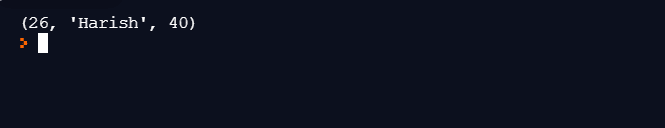As seen in the above code, the data types are different in the same tuples (string and int). We can also define Python tuples without the round brackets, but this method is not a conventional practice, and one should avoid it. Using python tuples without the brackets is called tuple packing.

### Special Case - Tuple with a Single Value

A particular case arises when the programmer wants to initialize a python tuple with a single number only. As a learner and a beginner, this is an essential case while studying for the PCEP exam. A single value in a tuple can only initialize by ending the value with a comma as follows:

``````tuples = (98,)
print(tuples)
``````

Output:The above output does not satisfy the arguments, though. Is it essential to put the comma at the end? For confirmation, let's use the "type" function and observe the output:

``````tuples = (98,)
print(type(tuples))
``````

Output: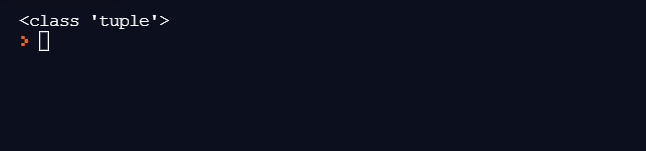If you are wondering what would be the output when we omit the last comma, don't hold yourself! Let's tweak the code to see why that comma is so essential. Execute the following code and observe the output:

``````tuples = (98)
print(type(tuples))
``````

Output: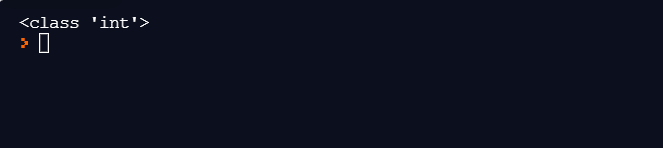Since there was a number inside the brackets, Python realized it as an "int. " For string, the output would come as a string. Therefore, always be careful when initializing a tuple with a single number to avoid errors later in the program.

### Properties of Python Tuples

The following section demonstrates various properties exhibited by the tuples with simple examples.

#### Duplicate Values in Python

Tuples in Python also allow duplicate values, unlike sets that have a dedicated post on ToolsQA.

``````tuples = (26, 40, 40)
print(tuples)
``````

Output: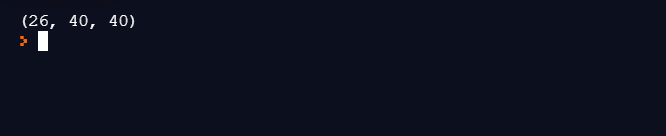As seen, there are two 40 in the tuple, which is allowed.

#### Python Tuples are Ordered

Python tuples are also ordered, i.e., they follow a specific order of indexing starting from the 0th index. It helps in the traversal and slicing of tuples easily.

The following code would work fine to print the first output of the following tuple:

``````tuples = (26, 40, 40)
print(tuples)
``````

Output:Similarly, other index values can be retrieved and/or used for other purposes in the Python program. Tuples also allow indexing with negative indexes. A negative index of -1 would signify the last element while -2 the second last. It is similar to Python lists.

#### Python Tuples are Immutable

As mentioned above, tuples are immutable in nature and hence do not allow any alterations. If you forcefully try to replace a value similar to Python lists, you may encounter the following error: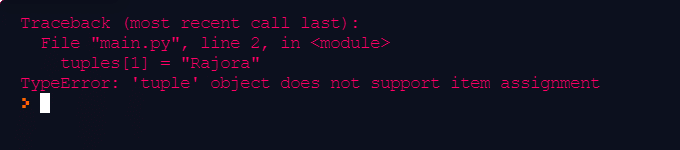The above error corresponds to the assignment operations. For other operations, you will face different errors.

### Operations on Python Tuples

Python tuples refrain from change but allow us to initialize a new tuple using existing tuples. Although, one should avoid such a repeated operation in Python as it takes unnecessary memory and might slow down the program's overall execution.

The following code assigns a new tuple based on the two existing tuples:

``````tuples1 = (26, 35, 60)
tuples2 = (16, 25, 50)
tuples3 = tuples1 + tuples2
print(tuples3)
``````

Output: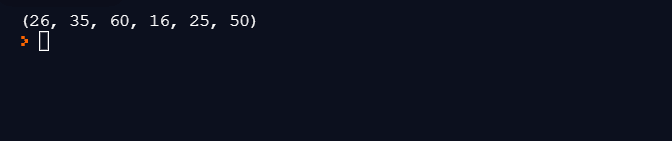The new tuple initializes as the concatenated version of both the tuples (tuples1 and tuples2). It resembles a vector in the C++ programming language. The tuple has to be iterated behind the scenes and copied one by one into the new tuple. It is not a magical operation, and hence we should avoid it until extremely necessary.

Another operation that we can perform while using tuples is the multiplication operation which is a popular operation in Python. The multiplication of the containers (data structures) with a constant number repeats the same container constant times without creating a new container.

For example, the following code will transform a tuple by multiplying it by 2:

``````tuples1 = (26, 35, 60)
tuples1 = tuples1*2
print(tuples1)
``````

Output:The above operation works similar to copying a tuple by iterating it, which takes time and memory. Therefore, this operation too should be used carefully.

#### Slicing a Python Tuple

We can slice a tuple just the way we slice a python list. To slice a tuple, we make use of the ":" symbol as follows:

``````tuples = (98,12,45,23,76,79,34)
print((tuples[2:6]))
``````

Output:#### Tuples inside Tuples - Creating a Python Tuple Matrix

Similar to lists, we can also use python tuples to create a matrix by nesting a tuple inside another. A simple code demonstrating the same phenomenon is as follows:

``````tuples = (98,(12,45),23,(76,79),34)
print(tuples)
``````

Output:#### How to delete a Python Tuple - The Del Operator

We can not modify a python tuple. Hence, deleting a particular element is not possible. However, python does provide us with a method to delete the complete tuple when its usage or lifecycle completes. A generous idea to save precious memory and garbage collection. To delete a tuple, just pass it through the "del " method as follows:

``````del(tuple_name)
``````

The following make use of the "print " statement to verify the deletion of the tuple. Execute this code and observe the output:

``````tuples = (98,12,45,23,76,79,34)
del(tuples)
print(tuples)
``````

Output:As expected, the error is about the "tuple" not being defined as our "del" method deleted it.

#### Python Tuples with For Loops and The Membership Test

Similar to the Python lists, we can also use the tuples with "for" loops along with the "in" operator to iterate over elements inside the tuple. Let's use the following example to iterate over a Python tuple:

``````tuples = (98,12,45,23,76,79,34)
for i in tuples:
print(i)

``````

Output:Similarly, the membership test can also be used with the "in" operator to verify if the element is present in the tuple or not.

``````tuples = (98,12,45,23,76,79,34)
print(26 in tuples)
``````

Output: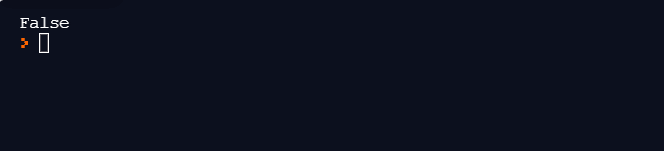A lot of these things might seem similar to the reader as they have been a part of the python lists chapter. Since we use both of them for data collection, they share some common functions, and the doubt is obvious. Practicing a mix of them is the best way to remember these functions. For your reference, a small table is also given below about common functions used with the tuples.

### Common Functions Used in Python Tuples

Along with the operators and methods discussed in the above section, we can use the following functions with tuples while constructing a python program:

Function Name Description Example
count To count the occurrence of an element in a tuple. tuple_name.count (‘element’)
index To find the first index of the element inside a tuple. tuple_name.index (‘element’)
len To find the length of the tuple. len (tuple_name)

### When to use Python Tuples?

Python tuples and python lists are almost similar in nature, with a few advantages of their own. They both confusing as to when we need to initialize a data collectable in the program. So, when should we go for a tuple and when to skip it?

A standard procedure of initializing a tuple is when the requirement is for immutable data. Tuples are immutable by nature. For a code that initializes a data container that guarantees to be constant throughout the program's life, tuples are the best choice. It will also come in handy security-wise as sensitive data would not change by any attacks and malpractices. Python tuples are also faster, and one can use them as key-pair value data as one can not change the data.

Another common culture developed among python developers is using tuples for heterogeneous data and lists for homogeneous data. Although there are no such protocols, some things take de facto standards due to similar acceptance on a large scale. Therefore, it is a good practice to use tuples when the data is heterogeneous.

## Key Takeaways:

• Python tuples are data collectable similar to python lists that save multiple data together.
• Tuples are immutable in nature, and once we initialize the data, we cannot alter it.
• Additionally, Tuples in Python are ordered and allows duplicate values to exist in the same tuple.
• One can operate the Tuples by pre-defined methods such as slicing, del, and count.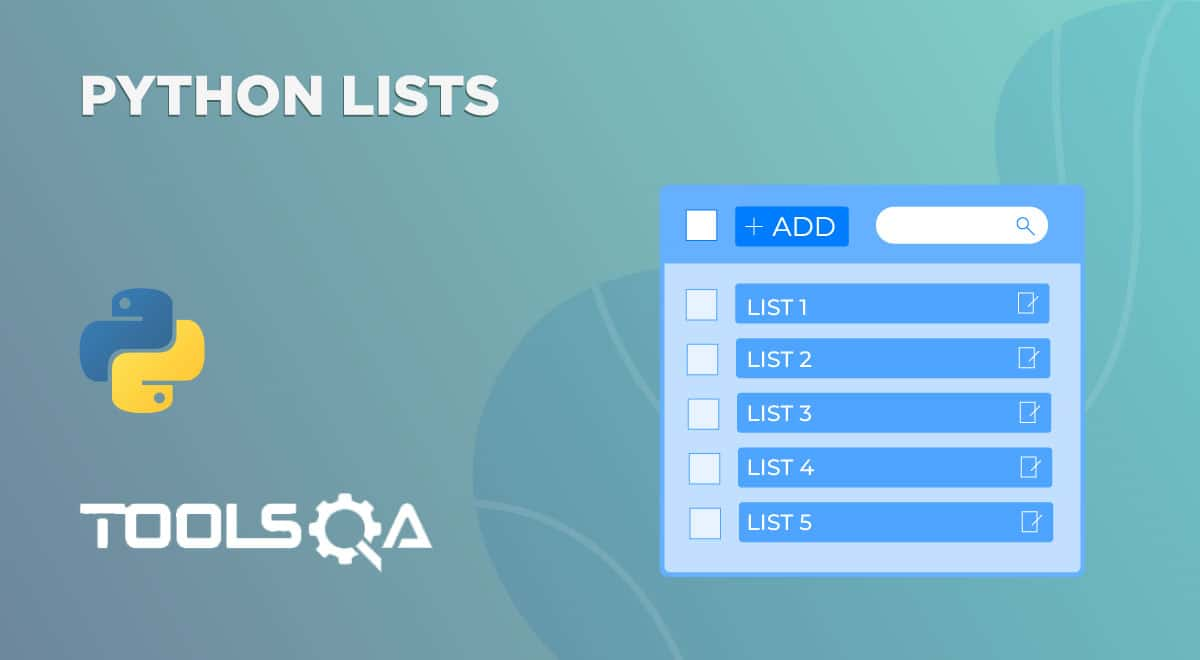ReviewersLakshay Sharma